Chemical Reactions CHAPTER 9 Chemical Reactions chemical reaction

• Slides: 56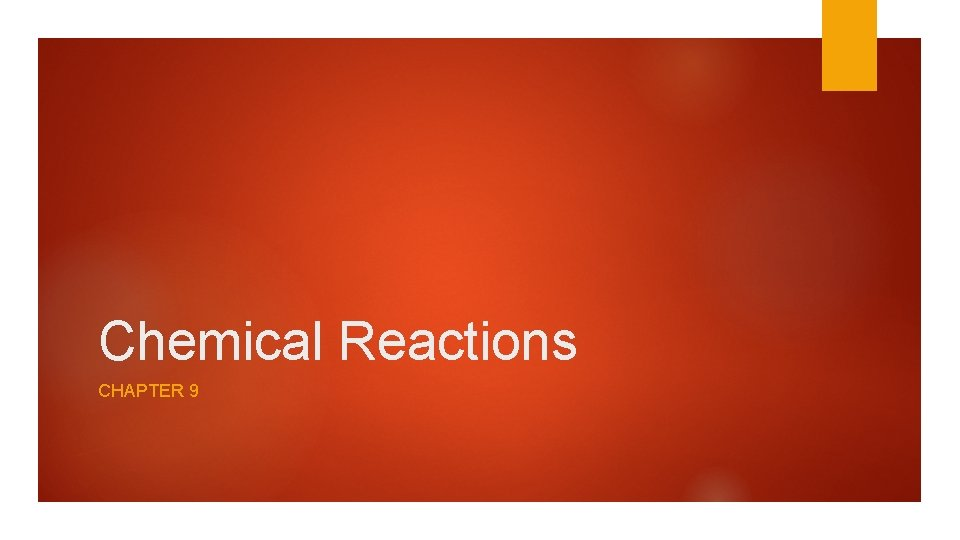Chemical Reactions CHAPTER 9Chemical Reactions chemical reaction- The process by which one or more substances are rearranged to form different substances Evidence that a chemical reaction may have occurred: – Change in temperature – Change in color – Odor – Gas bubbles – Appearance of a solid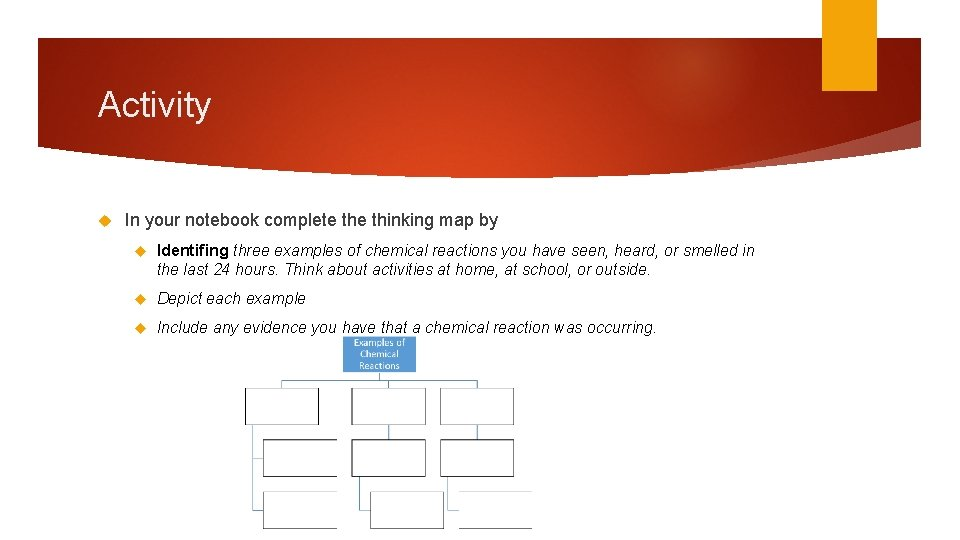Activity In your notebook complete thinking map by Identifing three examples of chemical reactions you have seen, heard, or smelled in the last 24 hours. Think about activities at home, at school, or outside. Depict each example Include any evidence you have that a chemical reaction was occurring.Representing Chemical Reactions Chemists use statements called equations to represent chemical reactions. • Reactants are the starting substances. • Products are the substances formed in the reaction.Activity Label the chemical state each symbol below identifies in a chemical equation. (s) _____________________________ (g) _____________________________ (aq) _____________________________ (l) _________________________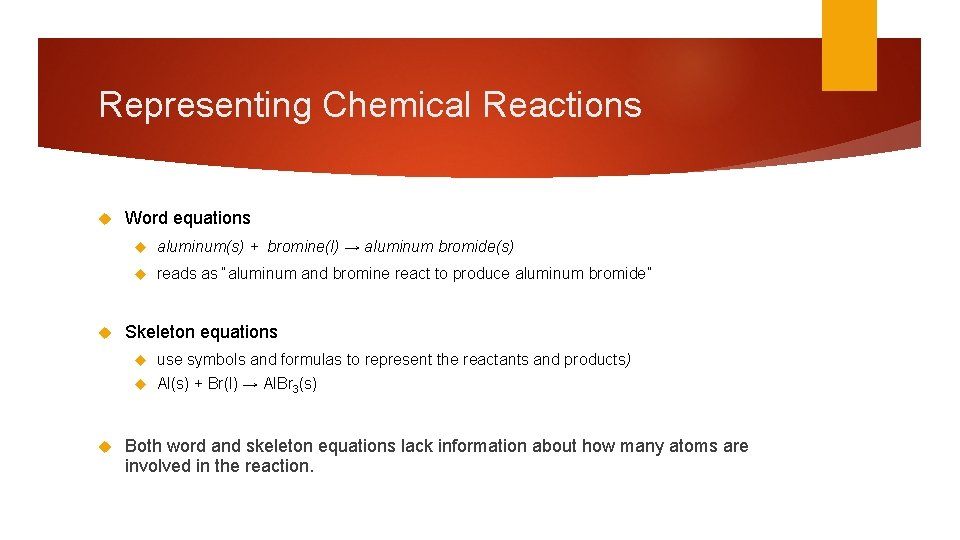Representing Chemical Reactions Word equations aluminum(s) + bromine(l) → aluminum bromide(s) reads as “aluminum and bromine react to produce aluminum bromide” Skeleton equations use symbols and formulas to represent the reactants and products) Al(s) + Br(l) → Al. Br 3(s) Both word and skeleton equations lack information about how many atoms are involved in the reaction.Representing Chemical Reactions Chemical equation- a statement that uses chemical formulas to show the identities and relative amounts of the substances involved in a chemical reaction.Activity In your notebook on the next page Recreate the following table Organize types of equations that can express a chemical reaction. In the second column, list the elements (words, coefficients, etc. ) that are used to create each equation. In the third column, rank each equation from 1 to 3, giving a 3 to the equation that provides the most information, and a 1 to the equation that provides the least information.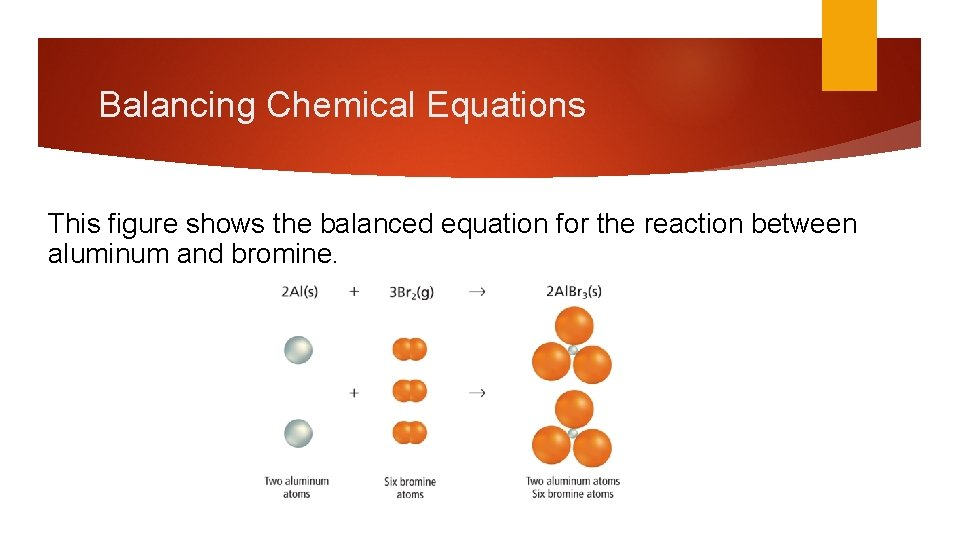Balancing Chemical Equations This figure shows the balanced equation for the reaction between aluminum and bromine.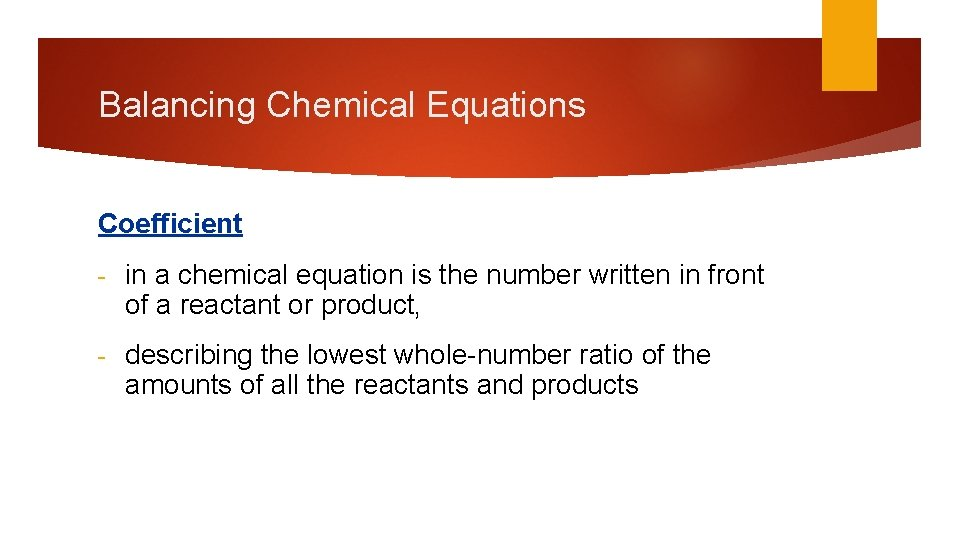Balancing Chemical Equations Coefficient - in a chemical equation is the number written in front of a reactant or product, - describing the lowest whole-number ratio of the amounts of all the reactants and productsBalancing Chemical Equations The most fundamental law in chemistry is the law of conservation of mass. • Balanced equations show this law.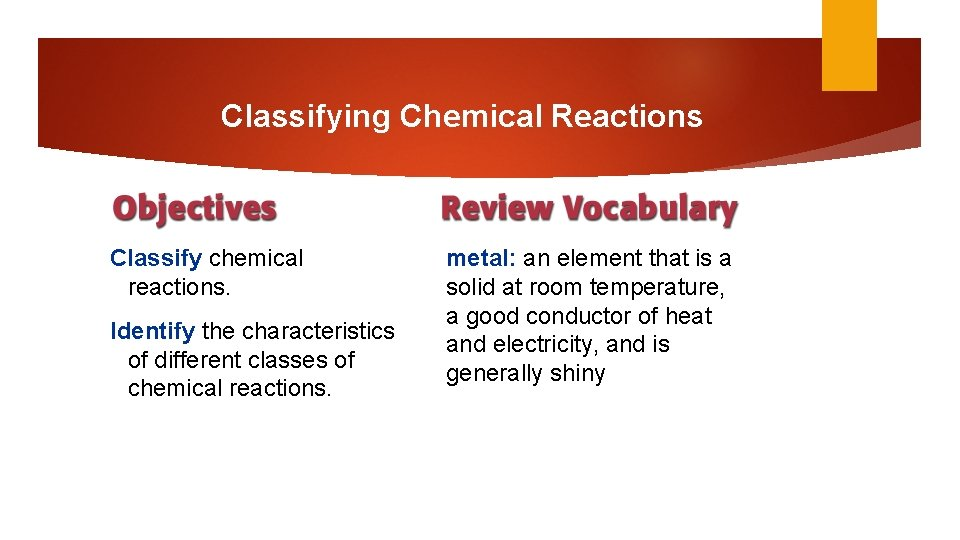Classifying Chemical Reactions Classify chemical reactions. Identify the characteristics of different classes of chemical reactions. metal: an element that is a solid at room temperature, a good conductor of heat and electricity, and is generally shiny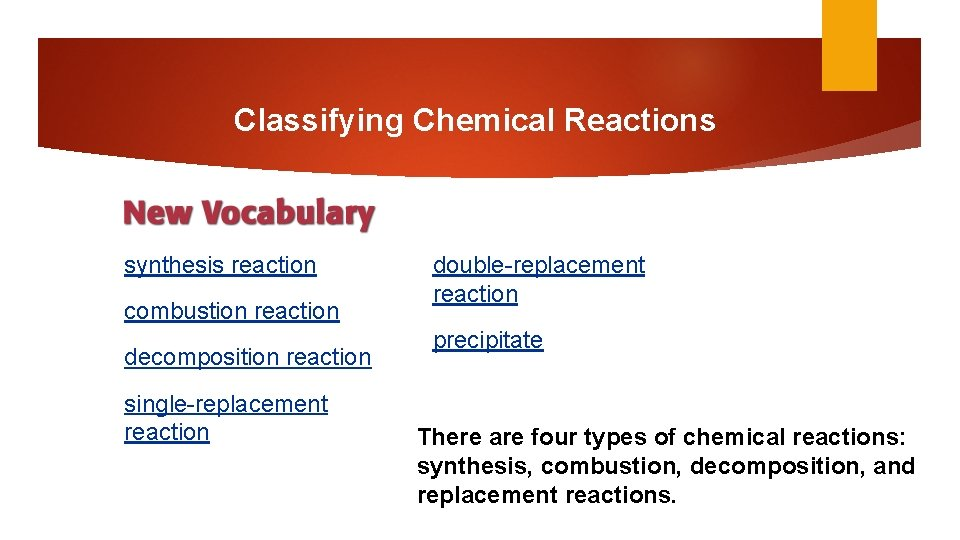Classifying Chemical Reactions synthesis reaction combustion reaction decomposition reaction single-replacement reaction double-replacement reaction precipitate There are four types of chemical reactions: synthesis, combustion, decomposition, and replacement reactions.Classifying Chemical Reactions Types of Chemical Reactions Chemists classify reactions in order to organize the many types. A synthesis reaction is a reaction in which two or more substances react to produce a single product. A + B → ABClassifying Chemical Reactions Types of Chemical Reactions (cont. ) When two elements react, the reaction is always a synthesis reaction.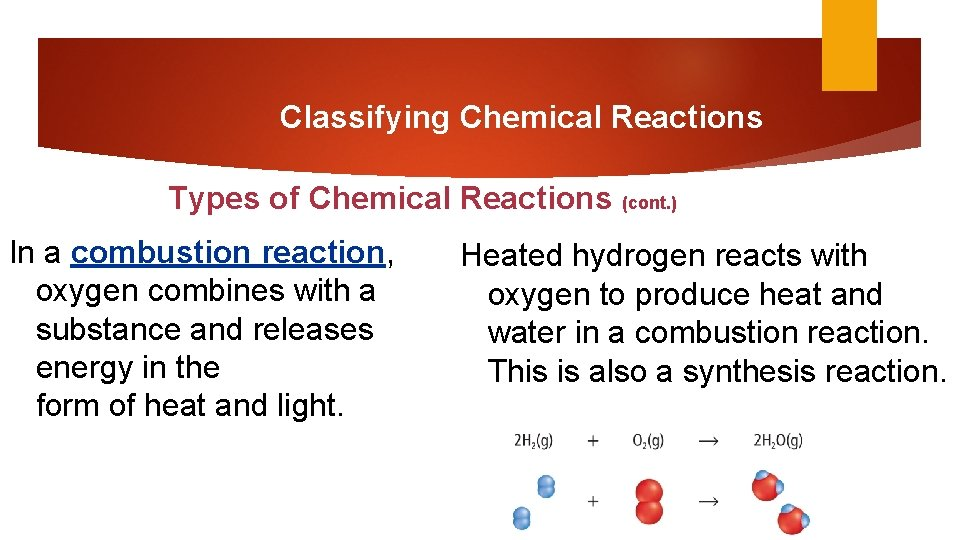Classifying Chemical Reactions Types of Chemical Reactions (cont. ) In a combustion reaction, oxygen combines with a substance and releases energy in the form of heat and light. Heated hydrogen reacts with oxygen to produce heat and water in a combustion reaction. This is also a synthesis reaction.Classifying Chemical Reactions Decomposition Reactions A decomposition reaction is one in which a single compound breaks down into two or more elements or new compounds. Decomposition reactions often require an energy source, such as heat, light, or electricity, to occur. AB → A + B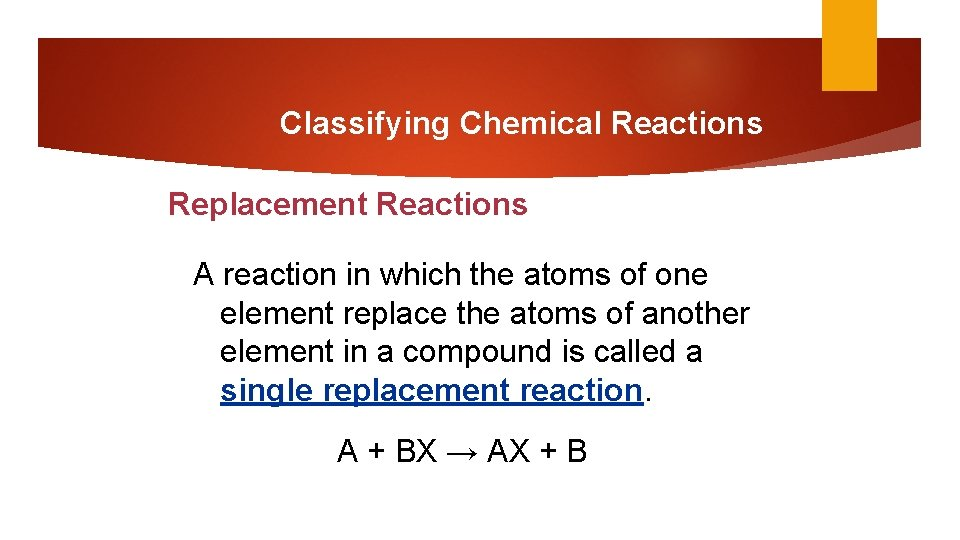Classifying Chemical Reactions Replacement Reactions A reaction in which the atoms of one element replace the atoms of another element in a compound is called a single replacement reaction. A + BX → AX + B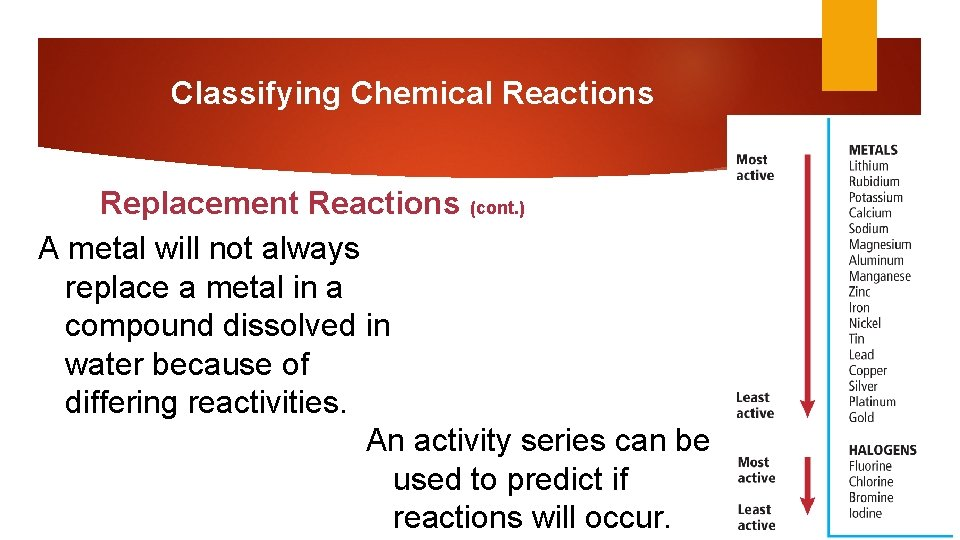Classifying Chemical Reactions Replacement Reactions (cont. ) A metal will not always replace a metal in a compound dissolved in water because of differing reactivities. An activity series can be used to predict if reactions will occur.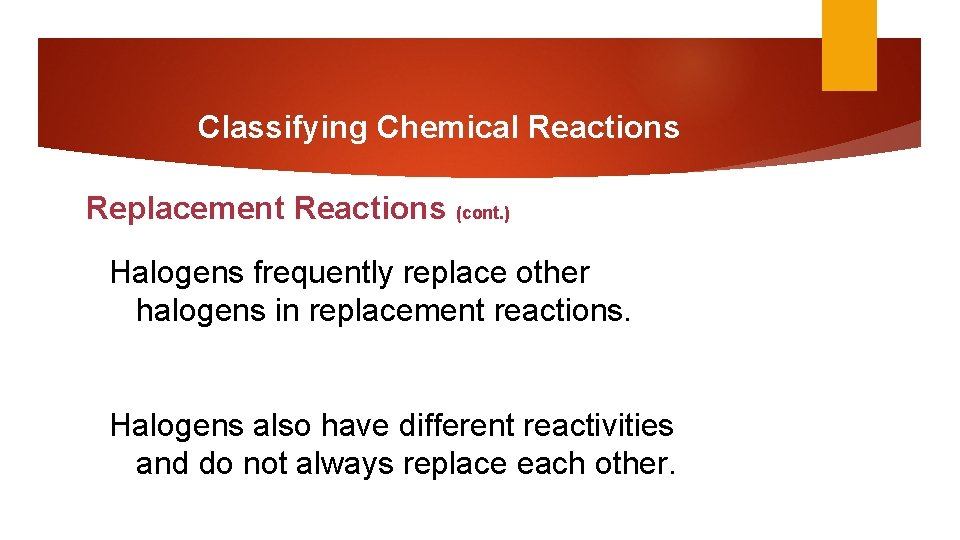Classifying Chemical Reactions Replacement Reactions (cont. ) Halogens frequently replace other halogens in replacement reactions. Halogens also have different reactivities and do not always replace each other.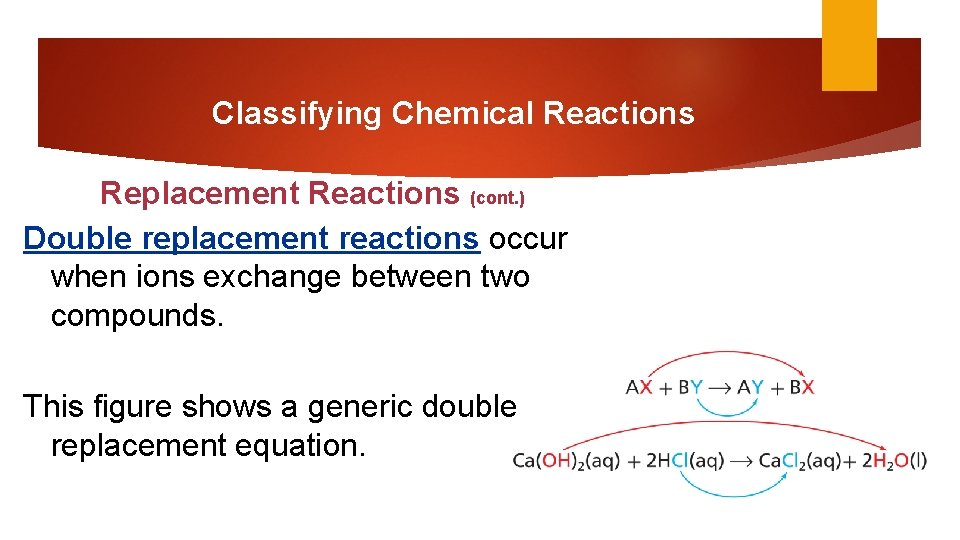Classifying Chemical Reactions Replacement Reactions (cont. ) Double replacement reactions occur when ions exchange between two compounds. This figure shows a generic double replacement equation.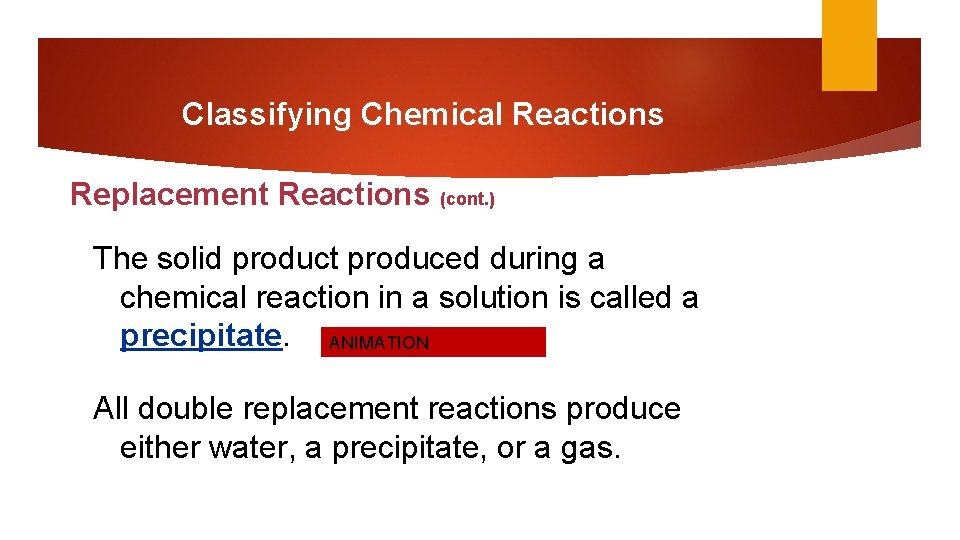Classifying Chemical Reactions Replacement Reactions (cont. ) The solid product produced during a chemical reaction in a solution is called a precipitate. ANIMATION All double replacement reactions produce either water, a precipitate, or a gas.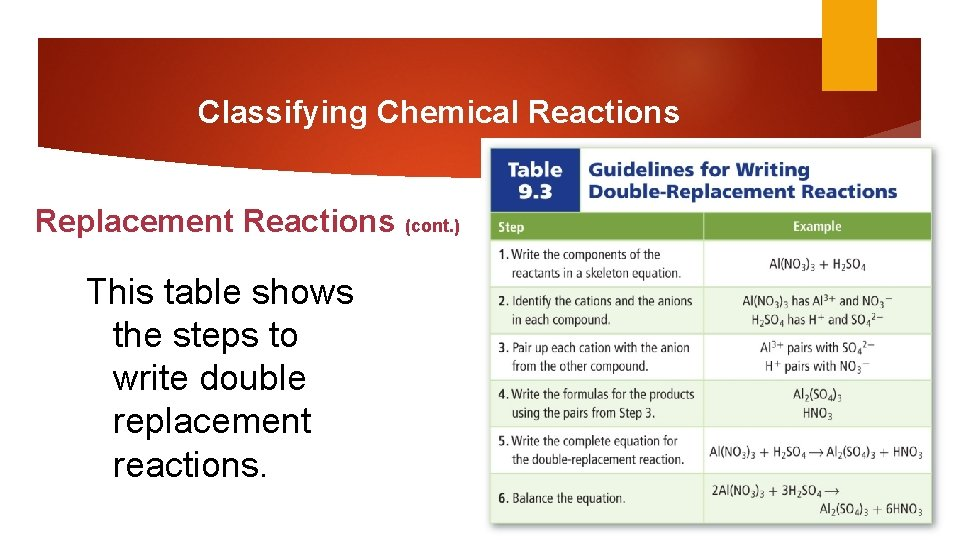Classifying Chemical Reactions Replacement Reactions (cont. ) This table shows the steps to write double replacement reactions.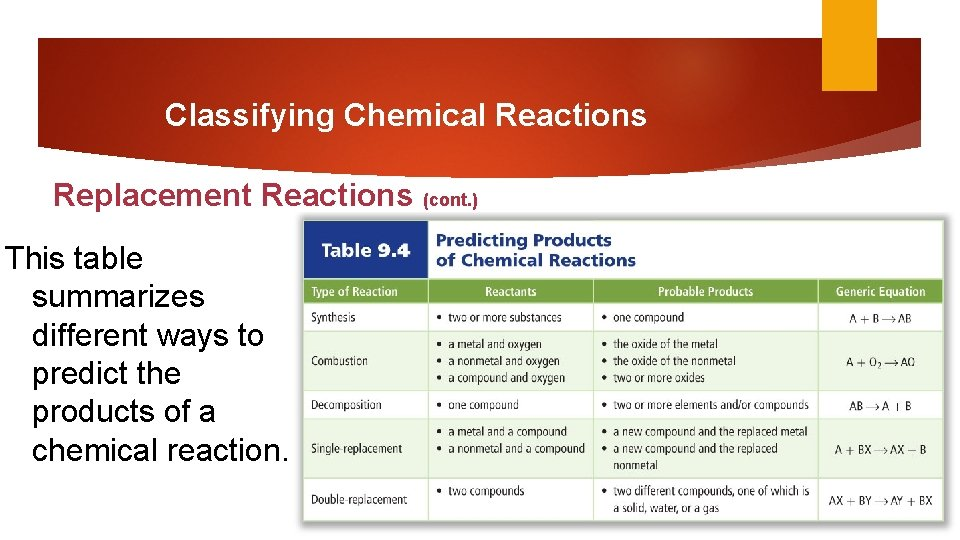Classifying Chemical Reactions Replacement Reactions (cont. ) This table summarizes different ways to predict the products of a chemical reaction.LAB Looking at some examples of chemical reactions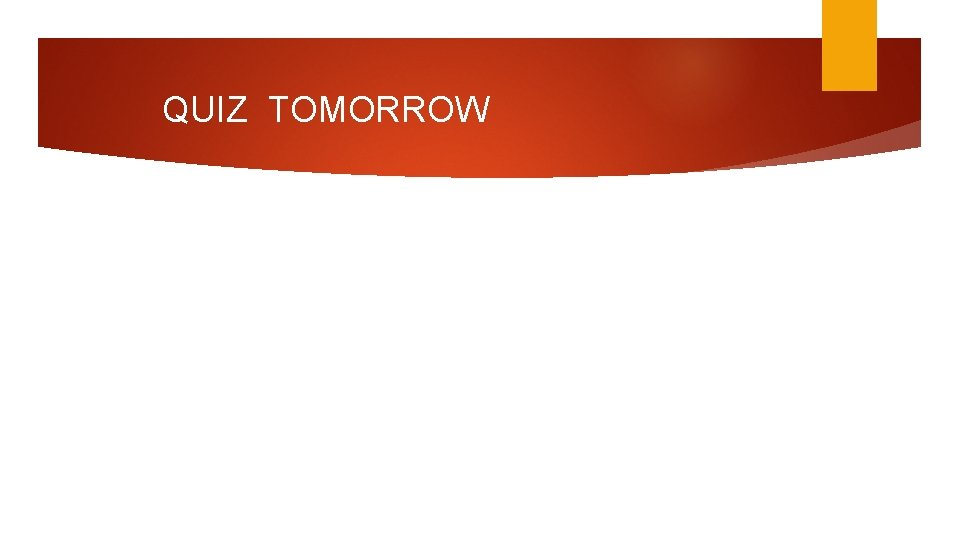QUIZ TOMORROWSection Check Which of the following is NOT one of the four types of reactions? A. deconstructive B. synthesis C. single replacement D. double replacementSection Check The following equation is what type of reaction? KCN(aq) + HBr(aq) → KBr(aq) + HCN(g) A. deconstructive B. synthesis C. single replacement D. double replacementReactions in Aqueous Solutions Describe aqueous solutions. Write complete ionic and net ionic equations for chemical reactions in aqueous solutions. Predict whether reactions in aqueous solutions will produce a precipitate, water, or a gas. solution: a uniform mixture that might contain solids, liquids, or gases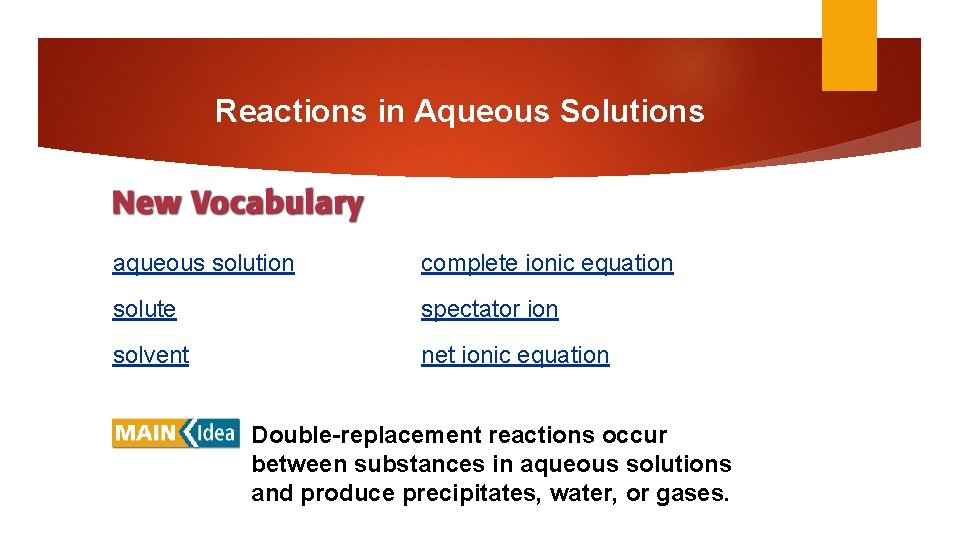Reactions in Aqueous Solutions aqueous solution complete ionic equation solute spectator ion solvent net ionic equation Double-replacement reactions occur between substances in aqueous solutions and produce precipitates, water, or gases.Reactions in Aqueous Solutions An aqueous solution contains one or more dissolved substances (called solutes) in water. The solvent is the most plentiful substance in a solution.Reactions in Aqueous Solutions (cont. ) Water is always the solvent in an aqueous solution. There are many possible solutes—sugar and alcohol are molecular compounds that exist as molecules in aqueous solutions. Compounds that produce hydrogen ions in aqueous solutions are acids.Reactions in Aqueous Solutions (cont. ) Ionic compounds can also be solutes in aqueous solutions. When ionic compounds dissolve in water, their ions separate in a process called dissociation.Reactions in Aqueous Solutions Types of Reactions in Aqueous Solutions When two solutions that contain ions as solutes are combined, the ions might react. If they react, it is always a double replacement reaction. Three products can form: precipitates, water, or gases.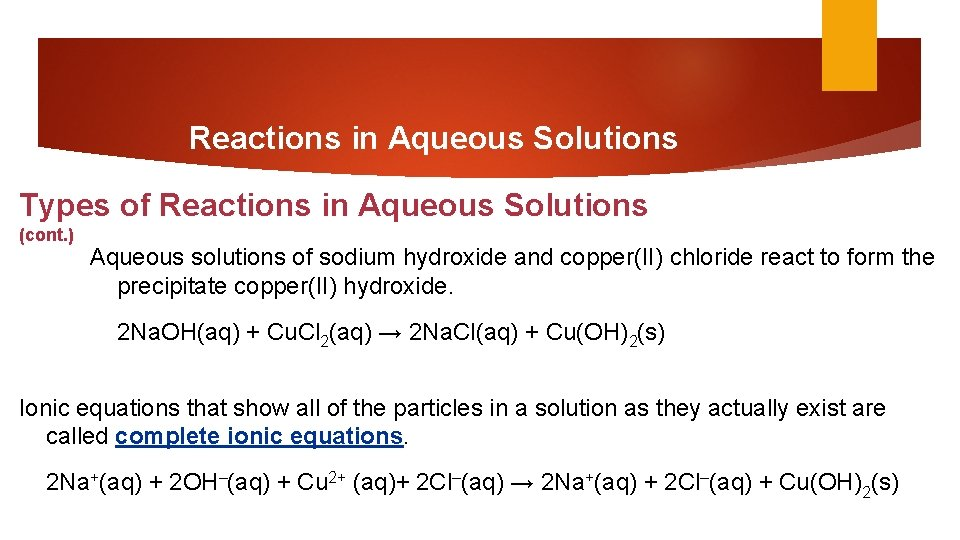Reactions in Aqueous Solutions Types of Reactions in Aqueous Solutions (cont. ) Aqueous solutions of sodium hydroxide and copper(II) chloride react to form the precipitate copper(II) hydroxide. 2 Na. OH(aq) + Cu. Cl 2(aq) → 2 Na. Cl(aq) + Cu(OH)2(s) Ionic equations that show all of the particles in a solution as they actually exist are called complete ionic equations. 2 Na+(aq) + 2 OH–(aq) + Cu 2+ (aq)+ 2 Cl–(aq) → 2 Na+(aq) + 2 Cl–(aq) + Cu(OH)2(s)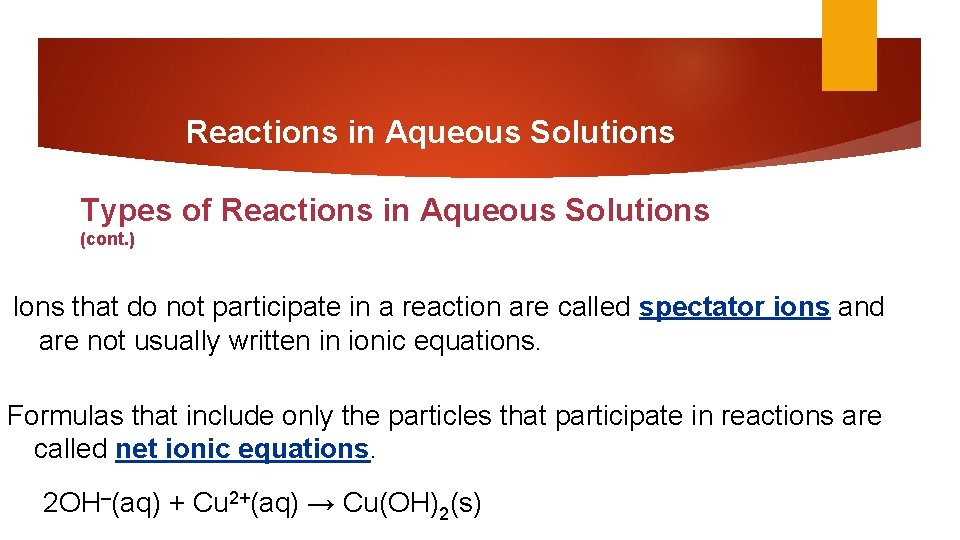Reactions in Aqueous Solutions Types of Reactions in Aqueous Solutions (cont. ) Ions that do not participate in a reaction are called spectator ions and are not usually written in ionic equations. Formulas that include only the particles that participate in reactions are called net ionic equations. 2 OH–(aq) + Cu 2+(aq) → Cu(OH)2(s)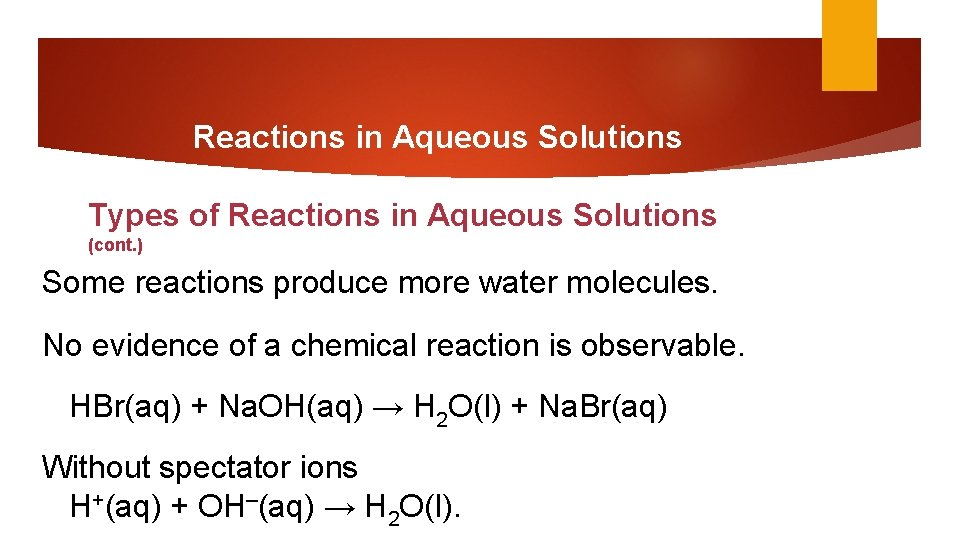Reactions in Aqueous Solutions Types of Reactions in Aqueous Solutions (cont. ) Some reactions produce more water molecules. No evidence of a chemical reaction is observable. HBr(aq) + Na. OH(aq) → H 2 O(l) + Na. Br(aq) Without spectator ions H+(aq) + OH–(aq) → H 2 O(l).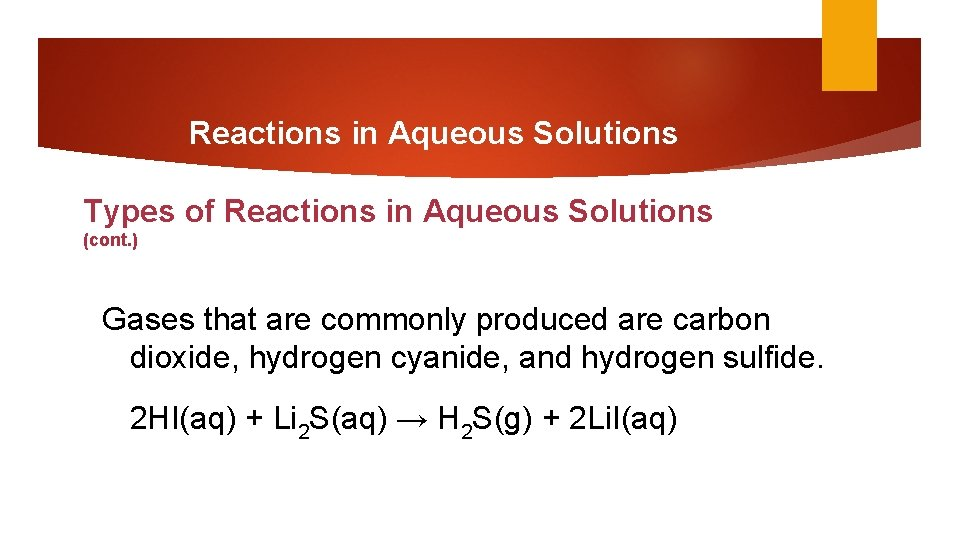Reactions in Aqueous Solutions Types of Reactions in Aqueous Solutions (cont. ) Gases that are commonly produced are carbon dioxide, hydrogen cyanide, and hydrogen sulfide. 2 HI(aq) + Li 2 S(aq) → H 2 S(g) + 2 Li. I(aq)Reactions in Aqueous Solutions Types of Reactions in Aqueous Solutions (cont. ) Another example is mixing vinegar and baking soda, which produces carbon dioxide gas. HCl(aq) + Na. HCO 3(aq) → H 2 CO 3(aq) + Na. Cl(aq) H 2 CO 3(aq) decomposes immediately. H 2 CO 3(aq) → H 2 O(l) + CO 2(g)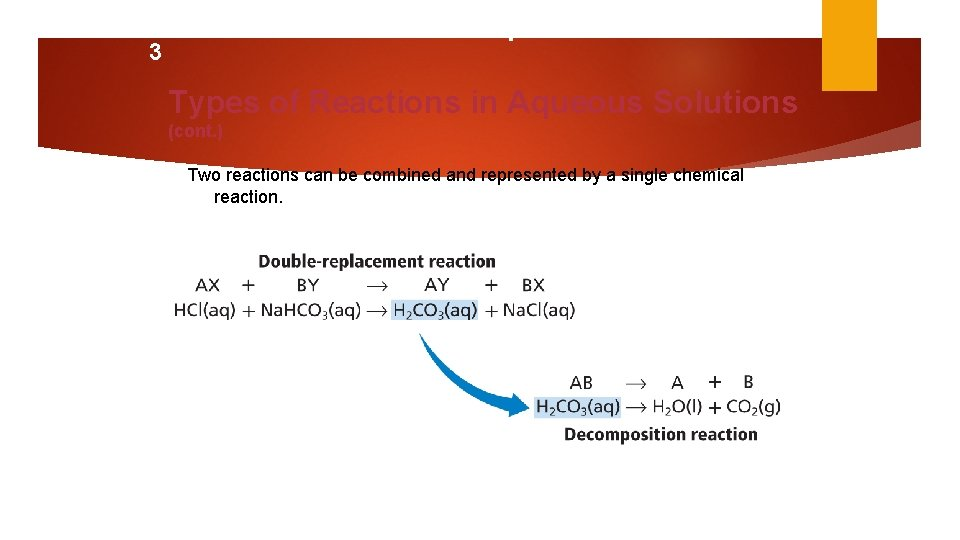9. SECTION 3 Reactions in Aqueous Solutions Types of Reactions in Aqueous Solutions (cont. ) Two reactions can be combined and represented by a single chemical reaction.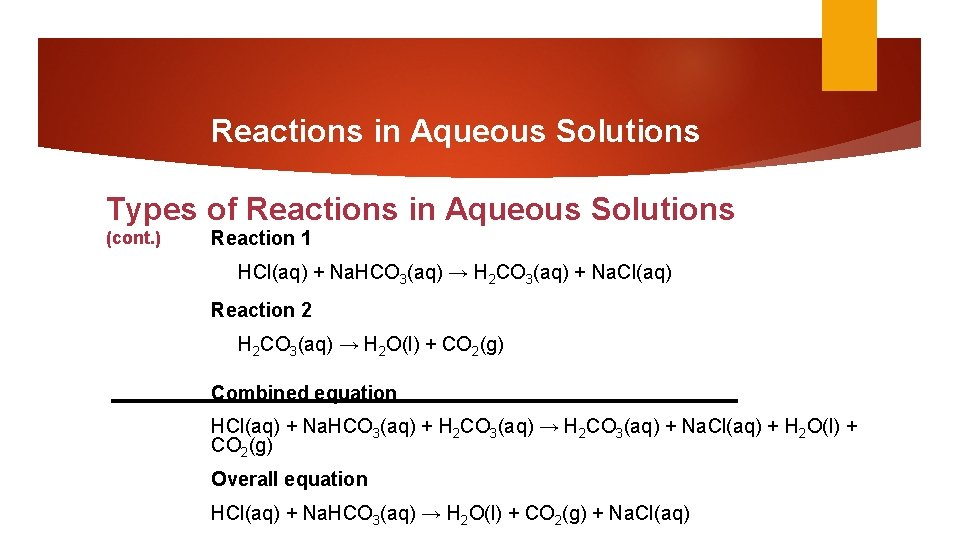Reactions in Aqueous Solutions Types of Reactions in Aqueous Solutions (cont. ) Reaction 1 HCl(aq) + Na. HCO 3(aq) → H 2 CO 3(aq) + Na. Cl(aq) Reaction 2 H 2 CO 3(aq) → H 2 O(l) + CO 2(g) Combined equation HCl(aq) + Na. HCO 3(aq) + H 2 CO 3(aq) → H 2 CO 3(aq) + Na. Cl(aq) + H 2 O(l) + CO 2(g) Overall equation HCl(aq) + Na. HCO 3(aq) → H 2 O(l) + CO 2(g) + Na. Cl(aq)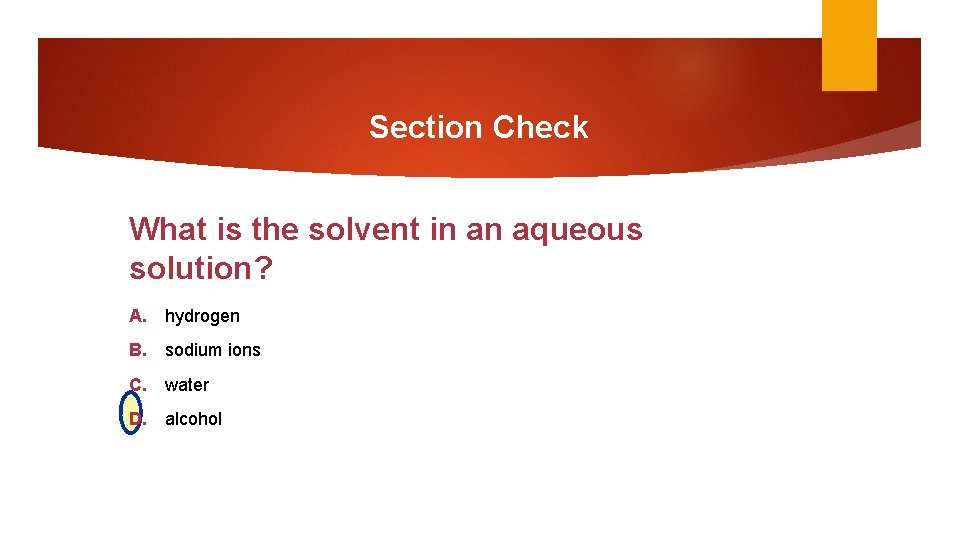Section Check What is the solvent in an aqueous solution? A. hydrogen B. sodium ions C. water D. alcoholSection Check An equation that includes only the particles that participate in a reaction is called: A. net ionic equation B. spectator ions C. complete ionic equation D. reduced ionic equation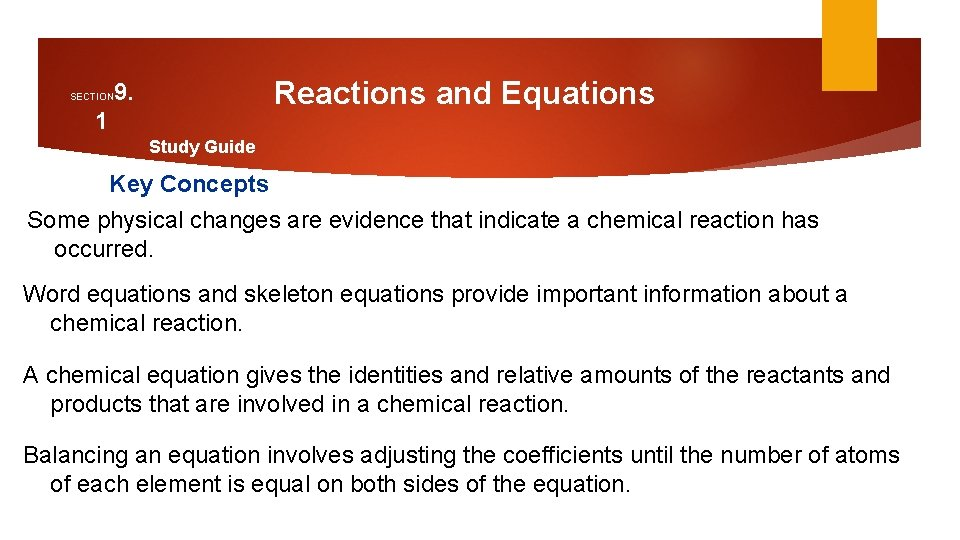Reactions and Equations 9. SECTION 1 Study Guide Key Concepts Some physical changes are evidence that indicate a chemical reaction has occurred. Word equations and skeleton equations provide important information about a chemical reaction. A chemical equation gives the identities and relative amounts of the reactants and products that are involved in a chemical reaction. Balancing an equation involves adjusting the coefficients until the number of atoms of each element is equal on both sides of the equation.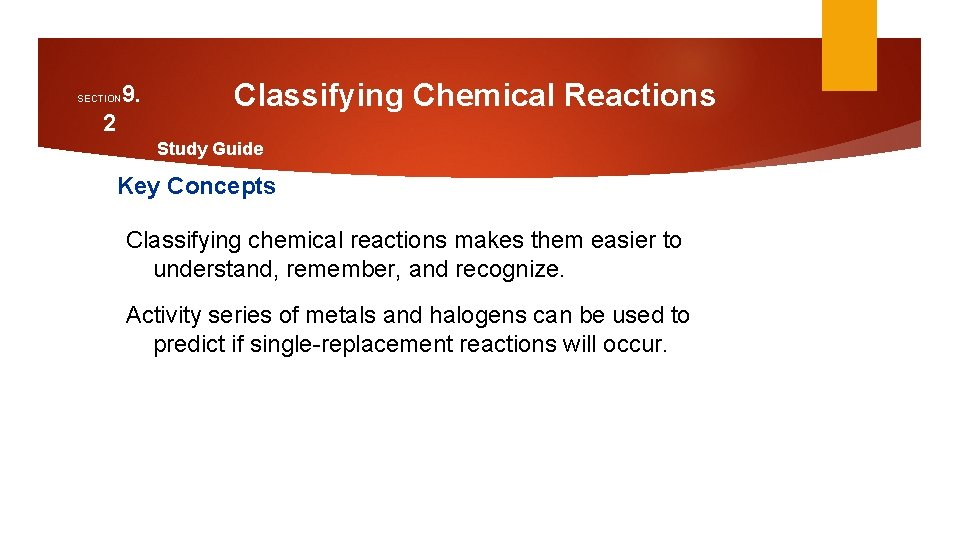9. SECTION 2 Classifying Chemical Reactions Study Guide Key Concepts Classifying chemical reactions makes them easier to understand, remember, and recognize. Activity series of metals and halogens can be used to predict if single-replacement reactions will occur.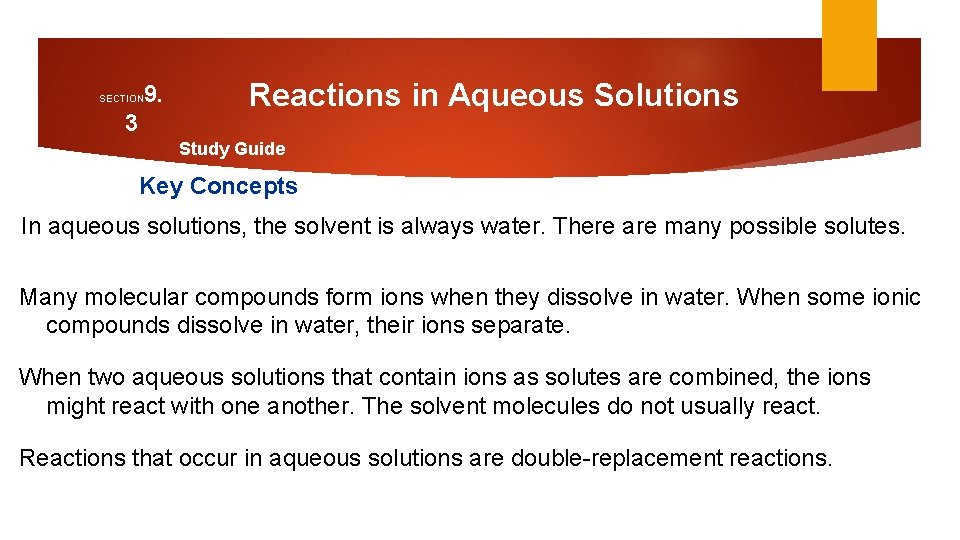9. SECTION 3 Reactions in Aqueous Solutions Study Guide Key Concepts In aqueous solutions, the solvent is always water. There are many possible solutes. Many molecular compounds form ions when they dissolve in water. When some ionic compounds dissolve in water, their ions separate. When two aqueous solutions that contain ions as solutes are combined, the ions might react with one another. The solvent molecules do not usually react. Reactions that occur in aqueous solutions are double-replacement reactions.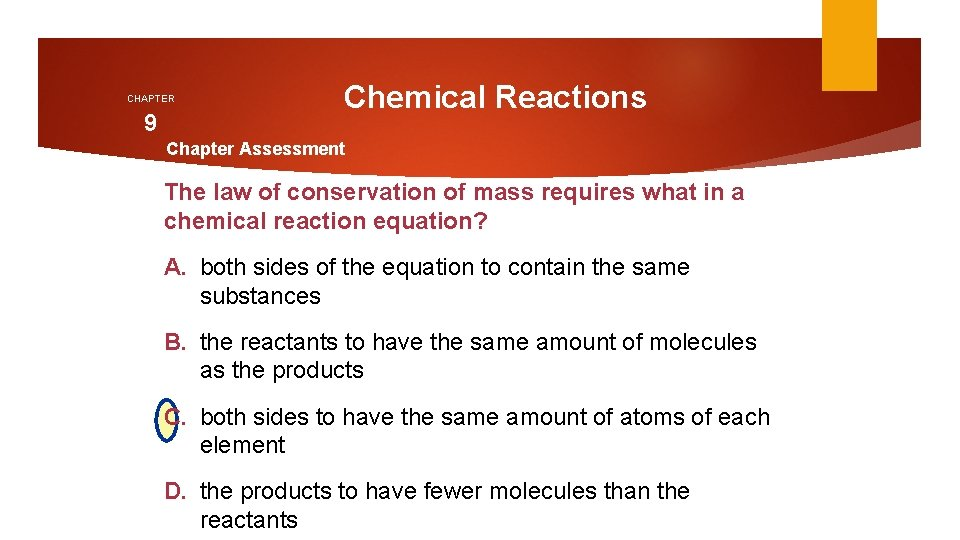CHAPTER 9 Chemical Reactions Chapter Assessment The law of conservation of mass requires what in a chemical reaction equation? A. both sides of the equation to contain the same substances B. the reactants to have the same amount of molecules as the products C. both sides to have the same amount of atoms of each element D. the products to have fewer molecules than the reactants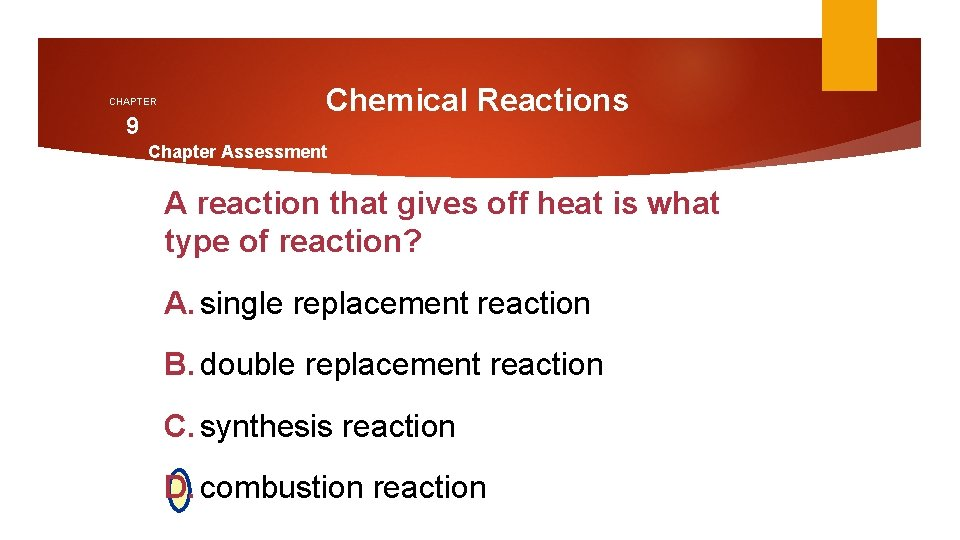CHAPTER 9 Chemical Reactions Chapter Assessment A reaction that gives off heat is what type of reaction? A. single replacement reaction B. double replacement reaction C. synthesis reaction D. combustion reaction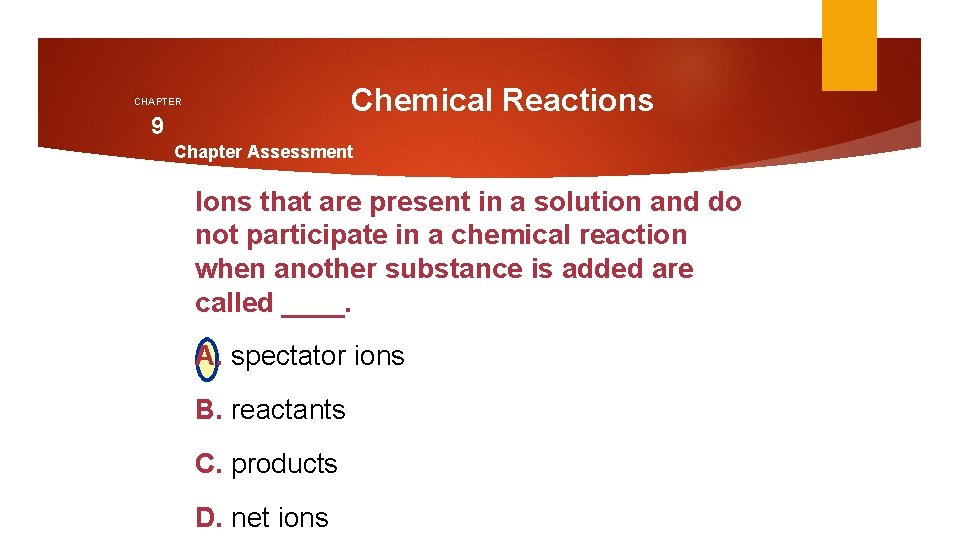Chemical Reactions CHAPTER 9 Chapter Assessment Ions that are present in a solution and do not participate in a chemical reaction when another substance is added are called ____. A. spectator ions B. reactants C. products D. net ionsCHAPTER 9 Chemical Reactions Chapter Assessment A double replacement reaction produces all of the following except ____. A. gases B. solids C. light D. water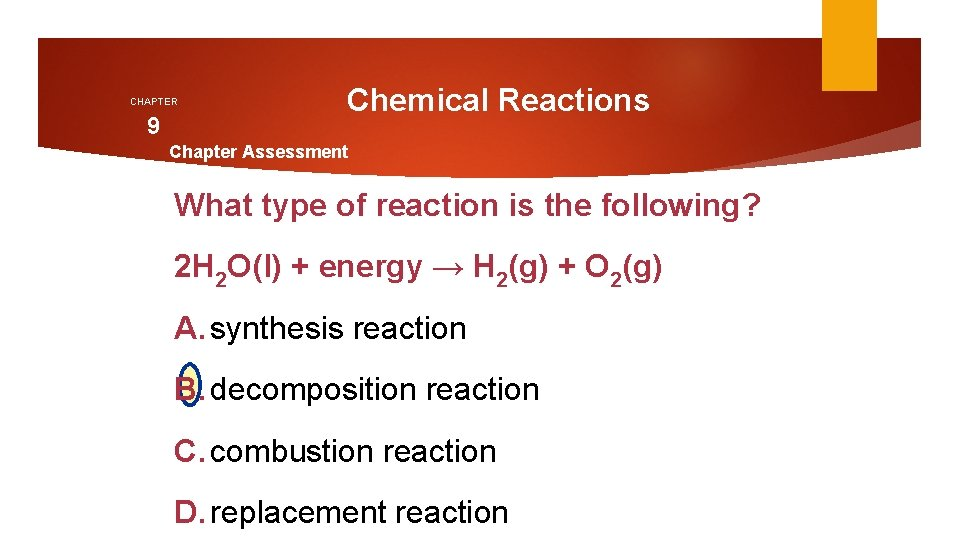CHAPTER 9 Chemical Reactions Chapter Assessment What type of reaction is the following? 2 H 2 O(l) + energy → H 2(g) + O 2(g) A. synthesis reaction B. decomposition reaction C. combustion reaction D. replacement reaction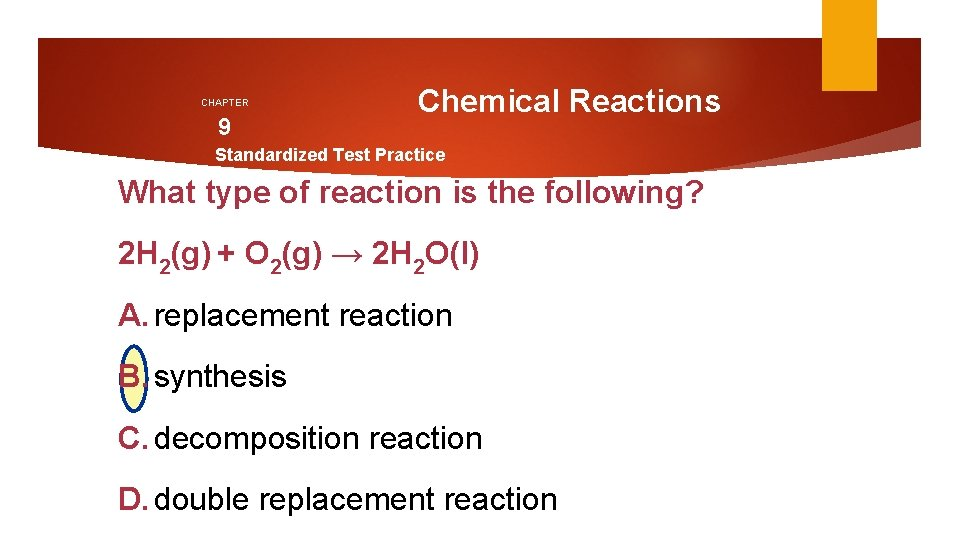CHAPTER 9 Chemical Reactions Standardized Test Practice What type of reaction is the following? 2 H 2(g) + O 2(g) → 2 H 2 O(l) A. replacement reaction B. synthesis C. decomposition reaction D. double replacement reaction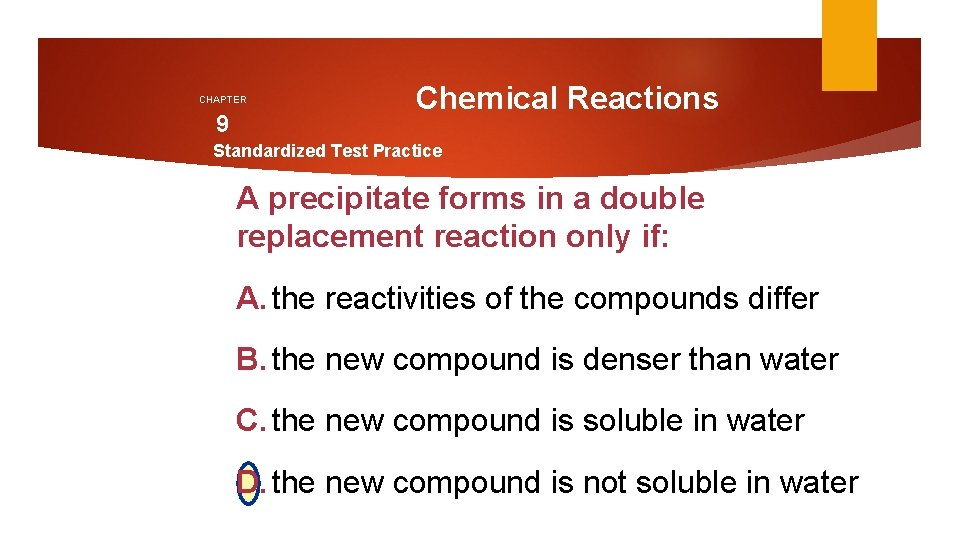CHAPTER 9 Chemical Reactions Standardized Test Practice A precipitate forms in a double replacement reaction only if: A. the reactivities of the compounds differ B. the new compound is denser than water C. the new compound is soluble in water D. the new compound is not soluble in water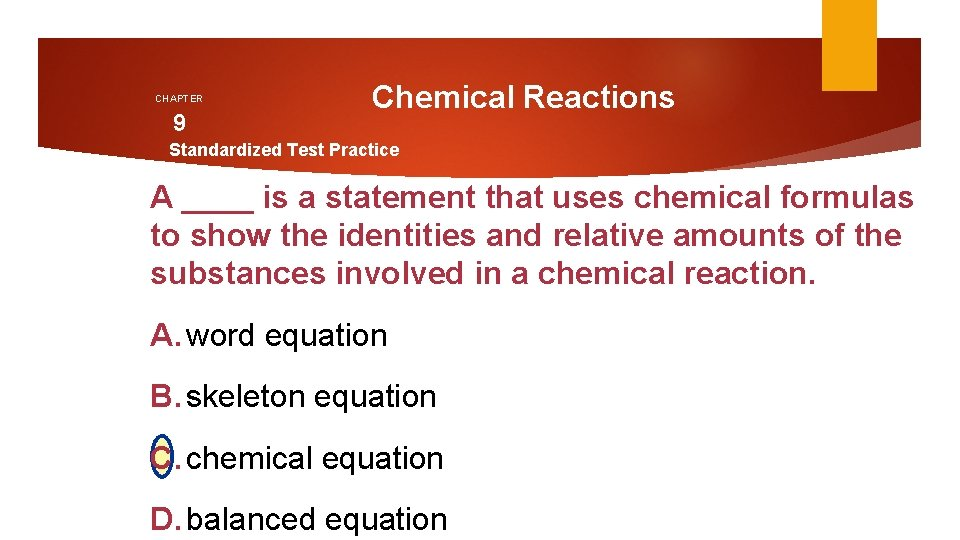CHAPTER 9 Chemical Reactions Standardized Test Practice A ____ is a statement that uses chemical formulas to show the identities and relative amounts of the substances involved in a chemical reaction. A. word equation B. skeleton equation C. chemical equation D. balanced equationCHAPTER 9 Chemical Reactions Standardized Test Practice Predict the type of reaction. Li. Br 2 (aq) + 2 Na. OH (aq) → ____ A. synthesis reaction B. combustion reaction C. single replacement reaction D. double replacement reaction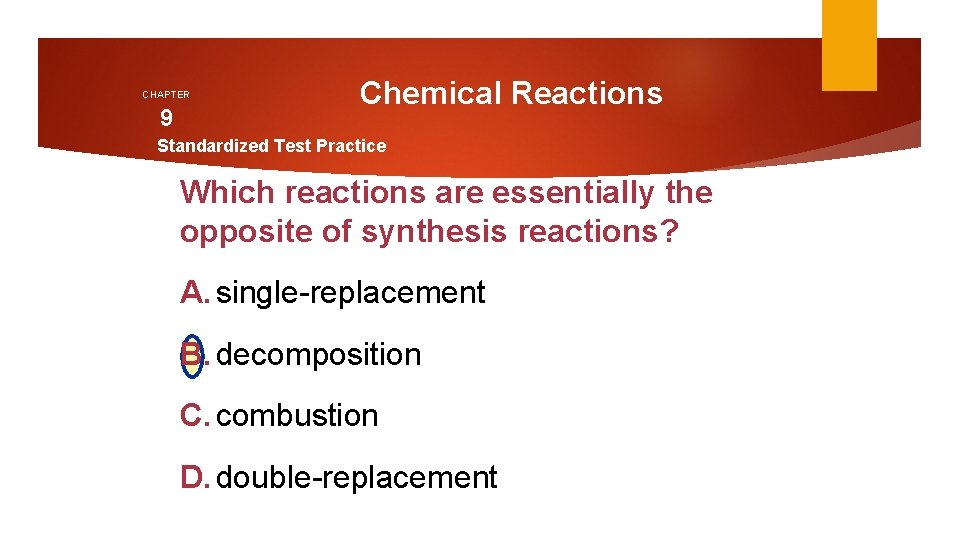CHAPTER 9 Chemical Reactions Standardized Test Practice Which reactions are essentially the opposite of synthesis reactions? A. single-replacement B. decomposition C. combustion D. double-replacement